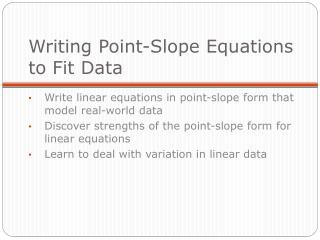DownloadDownload PresentationWriting Point-Slope Equations to Fit Data

# Writing Point-Slope Equations to Fit Data

Télécharger la présentation## Writing Point-Slope Equations to Fit Data

- - - - - - - - - - - - - - - - - - - - - - - - - - - E N D - - - - - - - - - - - - - - - - - - - - - - - - - - -
##### Presentation Transcript

1. Writing Point-Slope Equations to Fit Data Write linear equations in point-slope form that model real-world data Discover strengths of the point-slope form for linear equations Learn to deal with variation in linear data

2. In this lesson you will practice modeling data that have a linear pattern with the point-slope form of a linear equation. • You can then decide if using the point-slope form is more efficient than the intercept form.

3. Life Expectancy Page 248 Materials Needed Graph paper

4. This table shows the relationship between the number of years a person might be expected to live and the year he or she was born. • Life expectancy is a prediction that is very useful in professions like medicine and insurance.

5. Step 1: Choose one column of life expectancy data—female, male, or combined. Let x represent birth year, and let y represent life expectancy in years. Graph the data points. Step 2: Choose two points on your graph so that a line through them closely reflects the pattern of all the points on the graph. Use the two points to write the equation of this line in point-slope form. • Each group will work with either Female, Male or Combined Data

6. Each group will work with either Female, Male or Combined Data Step 3: Graph the line with your data points. Does it fit the data? Step 4: Use your equation to predict the life expectancy of a person who will be born in 2022.

7. Step 5: Compare your prediction from Step 4 to the prediction that another group made analyzing the same data. Are your predictions the same? Are they close? Explain why it’s possible to make different predictions from the same data. Step 6: Compare the slope of your line of fit to the slopes that other groups found working with different data sets. What does the slope for each data set tell you?

8. Step 7: As a class, select one line of fit that you think is the best model for each column of data—female, male, and combined. Graph all three lines on the same set of axes. Is it reasonable for the line representing the combined data to lie between the other two lines? Explain why or why not. Step 8: How does the point-slope method of finding a line compare to the intercept-form method you learned about in Lesson 4.2? What are the strengths and weaknesses of each method?

9. Summarize how you can fit a point-slope line to linear data. • How will you make sure that it fits the data you have graphed?

10. Writing Point-Slope Equations to Fit Data • Complete problems 1 and 2. • Then each group will be assigned one problem from 4, 5, or 6 to complete.